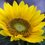# NMTC Problem

$\dfrac{x^3}{z^3+x^2y}+\dfrac{y^3}{x^3+y^2z}+\dfrac{z^3}{y^3+z^2x}\geq \dfrac{3}{2}$

Given that $x,y$ and $z$ are positive reals, prove the inequality above.Note by Abdur Rehman Zahid
5 years, 4 months ago

This discussion board is a place to discuss our Daily Challenges and the math and science related to those challenges. Explanations are more than just a solution — they should explain the steps and thinking strategies that you used to obtain the solution. Comments should further the discussion of math and science.

When posting on Brilliant:

• Use the emojis to react to an explanation, whether you're congratulating a job well done , or just really confused .
• Ask specific questions about the challenge or the steps in somebody's explanation. Well-posed questions can add a lot to the discussion, but posting "I don't understand!" doesn't help anyone.
• Try to contribute something new to the discussion, whether it is an extension, generalization or other idea related to the challenge.

MarkdownAppears as
*italics* or _italics_ italics
**bold** or __bold__ bold
- bulleted- list
• bulleted
• list
1. numbered2. list
1. numbered
2. list
Note: you must add a full line of space before and after lists for them to show up correctly
paragraph 1paragraph 2

paragraph 1

paragraph 2

[example link](https://brilliant.org)example link
> This is a quote
This is a quote
    # I indented these lines
# 4 spaces, and now they show
# up as a code block.

print "hello world"
# I indented these lines
# 4 spaces, and now they show
# up as a code block.

print "hello world"
MathAppears as
Remember to wrap math in $$ ... $$ or $ ... $ to ensure proper formatting.
2 \times 3 $2 \times 3$
2^{34} $2^{34}$
a_{i-1} $a_{i-1}$
\frac{2}{3} $\frac{2}{3}$
\sqrt{2} $\sqrt{2}$
\sum_{i=1}^3 $\sum_{i=1}^3$
\sin \theta $\sin \theta$
\boxed{123} $\boxed{123}$

Sort by:

Here is a short solution I found:

$\sum_{cyc} \dfrac{x^4}{xz^3+x^3z} \geq \dfrac{(x^2+y^2+z^2)^2}{2(xz^3+x^3y+y^3z)}\geq \dfrac{3}{2}$

Hence it suffices to prove that

$(x^2+y^2+z^2)^2\geq 3(xz^3+x^3y+y^3z)$

Which is the famous Vasc's inequality

- 5 years, 4 months ago

Well, proving the Vasc Inequality might be slightly more difficult.

- 5 years, 4 months ago

To be exact Tremendously difficult

It does not yeild to most classical inequalities!

- 5 years, 4 months ago

Yes, I know. I doubt it can be quoted in contests either.

- 5 years, 4 months ago

I found this amazingly elegant proof on AoPS:
$\left(a^2 + b^2 + c^2\right)^2 - 3\left(a^3b + b^3c + c^3a\right) \\ = \frac {1}{2}\left( \left( a^{2} - 2ab + bc - c^{2} + ca\right) ^2 + \left(b^{2} - 2bc + ca - a^{2} + ab\right)^2 + \left( c^{2} - 2ca + ab - b^{2} + bc\right)^2 \right) \geq 0$

- 5 years, 4 months ago

Awesome!!! (speechless)

- 5 years, 4 months ago

This is basically the only relatively clean proof!

- 5 years, 4 months ago

Yep! Titu and Vasc killed this... =D

- 5 years, 4 months ago

- 5 years, 4 months ago

You really want my long, expanded solution?

- 5 years, 4 months ago

Yes :P

- 5 years, 4 months ago

- 5 years, 4 months ago

I still haven't seen your long and expanded solution Sharky :P

- 5 years, 2 months ago

It isnt very difficult that way. Try yourself expanding the whole inequality,without any denominators. Then work with AM-GM and Shur. (And post the expanded stuff)

- 5 years, 2 months ago